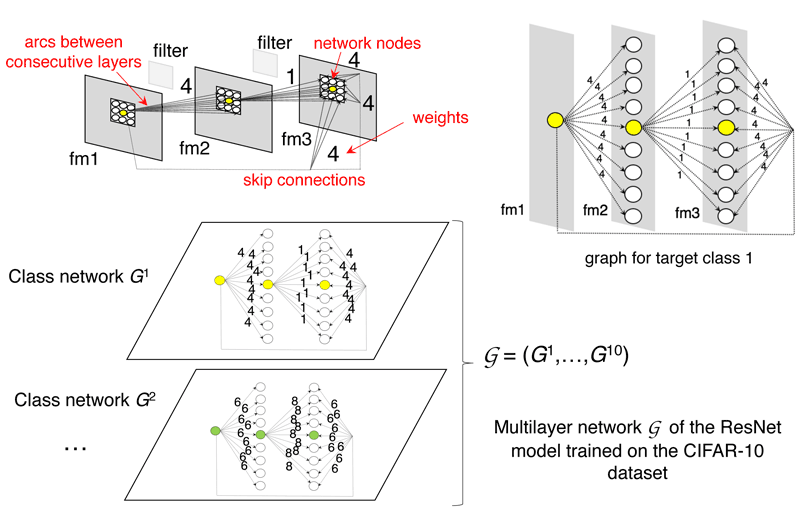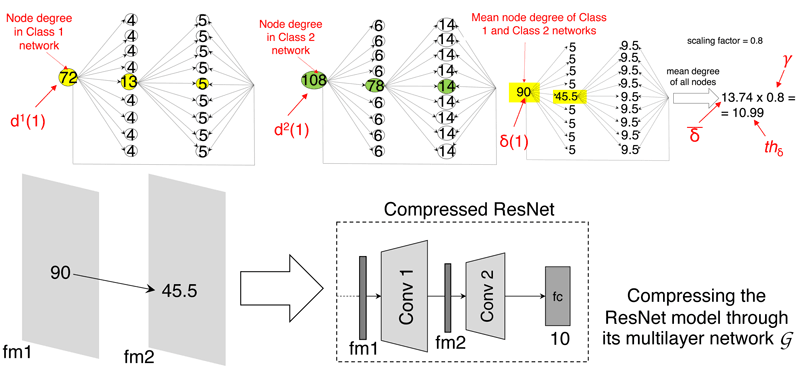# A Multilayer Network-Based Approach for Interpreting and Compressing Convolutional Neural Networks

by Alessia Amelio, Gianluca Bonifazi, Domenico Ursino and Luca Virgili (Polytechnic University of Marche)

We propose an approach to map a convolutional neural network (CNN) into a multilayer network. It allows the interpretability of the internal structure of deep learning architectures. Then, we use this representation to compress the CNN.

Researchers have recently become more aware of the necessity to scale back the size and complexity of deep neural networks. As a result, a number of techniques are being suggested to shrink the size of current networks without significantly impacting their performance. Exploring the many layers and components of a deep learning model is crucial in order to achieve this goal. In fact, one could pinpoint the most important components, the most relevant patterns and features, the information flow and so on. We want to make a contribution in this setting by proposing a new way of interpreting and exploring a CNN through a multilayer network representation of it, which is then used for compressing it .

We operate under the assumption that deep learning networks may be represented, analysed, explored and otherwise greatly supported by complex networks, particularly multilayer ones. Accordingly, we first introduce a method to transform deep learning networks into multilayer ones and then exploit the latter to explore and manipulate the former. Our study focuses on the CNN, which is a specific type of deep learning network widely adopted in different fields, especially computer vision; however, it can easily be extended to other kinds of deep learning networks. The multilayer network is a particular graph-based data structure composed of different layers. Each layer represents a graph with a specific type of connection among the nodes. Multilayer networks are a type of complex networks sophisticated enough to represent all the main components of a CNN. In fact, all the typical elements of a CNN (i.e. nodes, connections, filters, weights, etc.) can be represented through the basic components of a multilayer network (i.e. nodes, arcs, weights and layers). Once the representation of the CNN by the multilayer network has been obtained, the latter is adopted to explore and manipulate the former. To prove its potential, we use this representation to provide a method for removing unnecessary convolutional layers from a CNN. This method looks for layers in the CNN that can be pruned without significantly affecting the CNN performance and, if it finds any, it goes ahead and removes those layers, returning a new CNN .

More specifically, mapping the CNN into a multilayer network is performed in different steps (see Figure 1). In the first step, the CNN is trained from a database of images labelled with different target classes. Each image of the training set is forwarded through the network in order to predict its class. Then, for that image, the classification error between the predicted and target class is computed and back-propagated through the network for tuning its parameters. In the second step, each set of images of the dataset belonging to a target class is provided as input to the CNN in order to create a layer for the multilayer network. For each feature map of the CNN, each element becomes a node of the layer, and is linked to other nodes derived from the next feature maps according to their spatial adjacency. The weight of the arc between two nodes corresponds to the activation value associated with the first node in its feature map [2,3].Figure 1: Mapping a Residual Neural Network (ResNet) into a multilayer network for the CIFAR-10 dataset . Three convolutional layers of the ResNet produce three feature maps (fm1, fm2, fm3). Nodes of the multilayer network correspond to elements of the three feature maps. Arcs are created between adjacent nodes of subsequent feature maps. The weight of an arc between two nodes corresponds to the activation value of the first node in its feature map. There is one layer for each target class of the dataset, resulting in a multilayer network with ten layers (class networks).

We start from the assumption that nodes of the multilayer network with higher degree (defined for a node as the number of arcs crossing it) correspond to more informative areas of the feature maps of the CNN. Accordingly, the CNN is compressed through the following steps (see Figure 2). First, the degree of each node in each layer of the multilayer network is computed. Then, the total degree of each node over the different layers is calculated. Afterward, nodes with a total degree higher than a threshold are detected. In particular, the latter is computed as the mean degree of all nodes multiplied by a scaling factor. Finally, the only feature maps containing selected nodes are retained in the CNN, while the other ones are removed [2,3].Figure 2: Compressing the ResNet of Figure 1 when two target classes are considered . The two layers of the multilayer network corresponding to the target classes are reported on the top left and top mid of the figure (yellow and green coloured). The third graph on the top right contains the total degree of each node over the two layers. The value 10.99 of the threshold is obtained as the mean degree of all nodes, which is 13.74, multiplied by the scaling factor, which is 0.8. The only two nodes exceeding the threshold are those with mean degrees of 90 and 45.5. Since they are located on the first and second feature maps, fm1 and fm2, only the first and second convolutional layers will be retained in the CNN.

We adopted our approach for compressing two well-known CNNs, i.e. VGG  and ResNet , on different benchmark datasets in computer vision. The adopted datasets are: (i) MNIST, for the identification of handwritten digits from 0 to 9; (ii) CALTECH-101, for the recognition of objects belonging to 101 distinct classes; (iii) CIFAR-10; and (iv) CIFAR-100, for the recognition of objects belonging to 10 and 100 distinct classes, respectively. The obtained results show that our approach overcomes, in terms of different performance measures, another similar approach that uses a single-layer network for representing the CNN, as well as other approaches for compressing CNNs [2,3] proposed in the past literature.

This paper should not be considered as an endpoint but rather as a starting point for further research. A possible advancement in this direction is the development of a mechanism that allows the visualisation of the network to facilitate the possible interpretation of the compression result.

The approach described in this paper is the result of a collaboration between the Department of Engineering and Geology, University “G. d’Annunzio” Chieti-Pescara, Italy, and the Department of Information Engineering, Polytechnic University of Marche, Italy. A GitHub repository with the source code of the proposed approach is available at [L1].

[L1] https://github.com/lucav48/cnn2multilayer

References:
 A. Amelio, G. Bonifazi, E. Corradini, et al., “Mapping and compressing a convolutional neural network through a multilayer network,” presented at the 30th Symposium on Advanced Database System, Tirrenia (Pisa), Italy, June 19–22, 2022.
 A. Amelio, G. Bonifazi, E. Corradini, et al., “A multilayer network-based approach to represent, explore and handle convolutional neural networks,” Cognitive Computation, vol. 15, pp. 61–89, 2023.
 A. Amelio, G. Bonifazi, F. Cauteruccio, et al., “Representation and compression of Residual Neural Networks through a multilayer network based approach,” Expert Systems with Applications, vol. 215, 119391, 2023.

Luca Virgili, DII, Polytechnic University of Marche, Italy
This email address is being protected from spambots. You need JavaScript enabled to view it.

Alessia Amelio, InGeo, University “G. d’Annunzio” Chieti-Pescara, Italy
This email address is being protected from spambots. You need JavaScript enabled to view it.

Next issue: July 2023
Special theme:
"Eplainable AI"
Call for the next issue
Save